Hyperbolas, Logarithms and Exponentials

We have already seen than we can define the logarithm function as an integral of the rectangular hyperbola 1/x. This function is called the natural logarithm. We can expect that different hyperbolas (obtained multiplying the rectangular hyperbola by a number)results in different logarithms functions with the same properties.

This logarithms functions are called logarithm to the base b.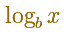We are going to explore the relation between this base b and the factor that multiply the hyperbola 1/x:We expect thatReplacing x by b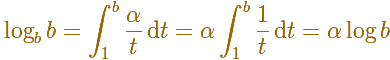Then

We get a very important relation between these logarithms functions:

Logarithms to the base b are obtained from natural logarithms by multiplying by a constant (and we can change the base of the logarithm very easily).

Then the graph of the function logarithm to the base b may be obtained from that of the natural logarithm function simply multiplying all ordinanates by the same factor. When b > 1 this factor is positive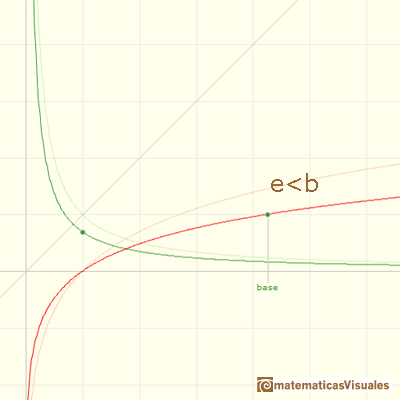An important particular case, decimal logarithm:When b < 1, this factor is negative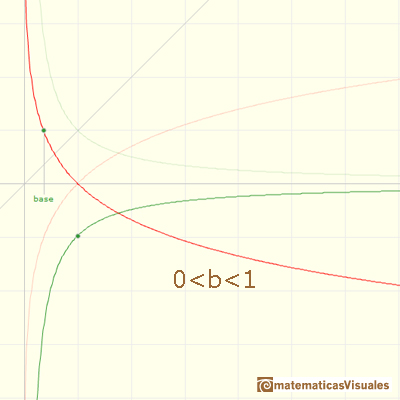EXPLORE

Explore the shape of different logarithms functions.

What is the relation between graph of the log to base b and the log to base 1/b?

It is easy to diferentiate the function logarithm to the base b(S. Lang, p. 173) Starting wihtwe can handle the other exponential functions a^x with arbitrary a > 0 by using a common rule for logarithms and exponents, namely for any numbers b, c we have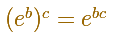We can define the exponential for every a > 0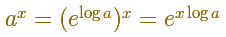For example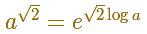And now we know how to calculate

Some properties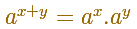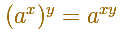Logarithms as exponents: the logarithm of a number is the exponent to which another fixed value, called the base, must be raised to get that number.The logarithm to base b es the inverse function of bx.

Just as the graph of the exponential function was obtained from that of the natural logarithm by reflection through the diagonal line y=x, the graph of these general exponential functions can be obtained by reflection of a logarithm function through the same line.EXPLORE

Explore the shape of different exponential functions. Some are increasing and some decreasing.

Is there a relation between the graph of the function ax and the graph of (1/a)x?

We can find the derivative by the chain rule: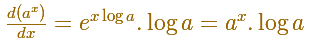Using the definition of the derivative: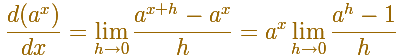Now we can calculate the limit

REFERENCES

A. I. Markushevich, Areas and Logarithms, D.C. Heath and Company, 1963.
Serge Lang, A First Course in Calculus, Third Edition, Addison-Wesley Publishing Company.
Tom M. Apostol, Calculus, Second Edition, John Willey and Sons, Inc.
Michael Spivak, Calculus, Third Edition, Publish-or-Perish, Inc.
Otto Toeplitz, The Calculus, a genetic approach, The University of Chicago Press, 1963.
Kenneth A. Ross, Elementary Analysis: The Theory of Calculus, Springer-Verlag New York Inc., 1980.We can study several properties of exponential functions, their derivatives and an introduction to the number e.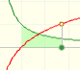Using the integral of the equilateral hyperbola we can define a new function that is the natural logarithm function.The natural logaritm can be defined using the integral of the rectangular hiperbola. In this page we are going to see an important property of this integral. Using this property you can justify that the logarithm of a product is the sum of the logarithms.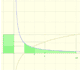The main property of a logarithm function is that the logarithm of a product is the sum of the logarithms of the individual factors.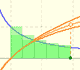The logarithm of the number e is equal to 1. Using this definition of the number e we can approximate its value.Constant e is the number whose natural logarithm is 1. It can be defined as a limit of a sequence related with the compound interest. Both definitions for e are equivalent.By increasing the degree, Taylor polynomial approximates the exponential function more and more.The Complex Exponential Function extends the Real Exponential Function to the complex plane.The complex exponential function is periodic. His power series converges everywhere in the complex plane.The Fundamental Theorem of Calculus tell us that every continuous function has an antiderivative and shows how to construct one using the integral.The Second Fundamental Theorem of Calculus is a powerful tool for evaluating definite integral (if we know an antiderivative of the function).As an introduction to Piecewise Linear Functions we study linear functions restricted to an open interval: their graphs are like segments.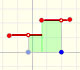A piecewise function is a function that is defined by several subfunctions. If each piece is a constant function then the piecewise function is called Piecewise constant function or Step function.A continuous piecewise linear function is defined by several segments or rays connected, without jumps between them.The integral of power functions was know by Cavalieri from n=1 to n=9. Fermat was able to solve this problem using geometric progressions.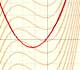If the derivative of F(x) is f(x), then we say that an indefinite integral of f(x) with respect to x is F(x). We also say that F is an antiderivative or a primitive function of f.The integral concept is associate to the concept of area. We began considering the area limited by the graph of a function and the x-axis between two vertical lines.Monotonic functions in a closed interval are integrable. In these cases we can bound the error we make when approximating the integral using rectangles.If we consider the lower limit of integration a as fixed and if we can calculate the integral for different values of the upper limit of integration b then we can define a new function: an indefinite integral of f.We can consider the polynomial function that passes through a series of points of the plane. This is an interpolation problem that is solved here using the Lagrange interpolating polynomial.